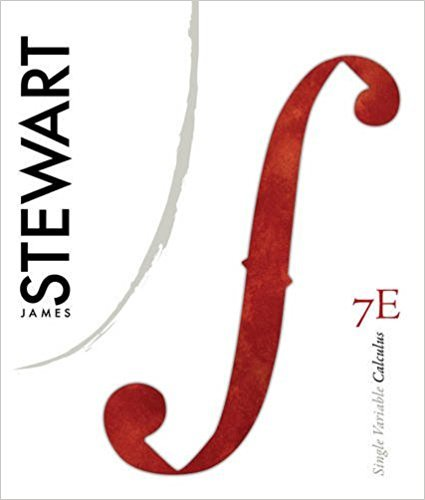×
×

# Solutions for Chapter 9: Single Variable Calculus 7th Edition## Full solutions for Single Variable Calculus | 7th Edition

ISBN: 9780538497831Solutions for Chapter 9

Solutions for Chapter 9
4 5 0 338 Reviews
20
5
##### ISBN: 9780538497831

Chapter 9 includes 25 full step-by-step solutions. This textbook survival guide was created for the textbook: Single Variable Calculus, edition: 7. This expansive textbook survival guide covers the following chapters and their solutions. Single Variable Calculus was written by and is associated to the ISBN: 9780538497831. Since 25 problems in chapter 9 have been answered, more than 5492 students have viewed full step-by-step solutions from this chapter.

Key Calculus Terms and definitions covered in this textbook
• Arctangent function

See Inverse tangent function.

• Augmented matrix

A matrix that represents a system of equations.

• Bounded above

A function is bounded above if there is a number B such that ƒ(x) ? B for all x in the domain of ƒ.

• Continuous function

A function that is continuous on its entire domain

• Directed angle

See Polar coordinates.

• Discriminant

For the equation ax 2 + bx + c, the expression b2 - 4ac; for the equation Ax2 + Bxy + Cy2 + Dx + Ey + F = 0, the expression B2 - 4AC

• Distance (in Cartesian space)

The distance d(P, Q) between and P(x, y, z) and Q(x, y, z) or d(P, Q) ((x ) - x 2)2 + (y1 - y2)2 + (z 1 - z 2)2

• equation of a parabola

(x - h)2 = 4p(y - k) or (y - k)2 = 4p(x - h)

• Exponential growth function

Growth modeled by ƒ(x) = a ? b a > 0, b > 1 .

• Half-angle identity

Identity involving a trigonometric function of u/2.

• Inferential statistics

Using the science of statistics to make inferences about the parameters in a population from a sample.

• Intercept

Point where a curve crosses the x-, y-, or z-axis in a graph.

• Length of an arrow

See Magnitude of an arrow.

• Ordered pair

A pair of real numbers (x, y), p. 12.

• Perihelion

The closest point to the Sun in a planet’s orbit.

• Product of a scalar and a vector

The product of scalar k and vector u = 8u1, u29 1or u = 8u1, u2, u392 is k.u = 8ku1, ku291or k # u = 8ku1, ku2, ku392,

• Residual

The difference y1 - (ax 1 + b), where (x1, y1)is a point in a scatter plot and y = ax + b is a line that fits the set of data.

• Sphere

A set of points in Cartesian space equally distant from a fixed point called the center.

• Variable

A letter that represents an unspecified number.

• Vertical line

x = a.

×